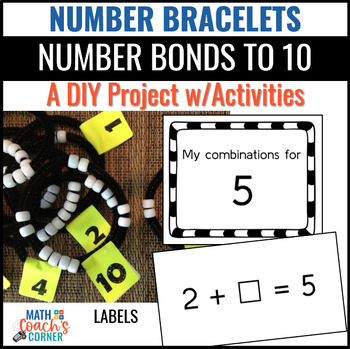DID YOU KNOW:
Seamlessly assign resources as digital activities

Learn how in 5 minutes with a tutorial resource. Try it Now

Learn More# Combinations to 10 with Number BraceletsGrade Levels
K - 2nd
Subjects
Standards
Resource Type
Formats Included
• PDF
Pages
26 pages

### Description

Number bracelets provide concrete practice for determining all the combinations of each number from 1 to 10. This ability to compose and decompose numbers in a variety of ways lays the foundation for fluency with addition and subtraction facts.

Teacher notes include:

• Instructions and labels for making the number bracelets
PLEASE NOTE: This is a DIY project and additional materials (chenille stems and pony beads) are required to make the bracelets
• Instructions for using the bracelets in a variety of ways in whole group, small group, and workstation settings with suggestions for differentiation
• Prompts for using with a math journal to record all combinations of the numbers from 1 to 10
• Missing addend equation cards for hands-on practice with the number bracelets

Download the preview for four pages of teacher notes describing all the activities.

Be sure to rate this product and provide feedback to earn TpT credits good toward the purchase of future products!

You Might Also Like…

Subitizing Cards: Perceptual and Conceptual, Print and Digital

Missing Part Flashcards Bundle: Print and Digital

Direct Modeling for 2-Digit Addition: Print and Digital Versions

Connect with Math Coach’s Corner

Be sure to follow my TpT store by clicking on the red ‘Follow Me’ next to my Seller picture to receive notifications of new products and upcoming sales. Visit my blog for K-5 math tips and freebies you can use tomorrow in your classroom. You can also find me on Facebook

and Twitter.

Terms of Use

Copyright © Donna Boucher. All rights reserved by author. This product is to be used by the original downloader only. Copying for more than one teacher, classroom, department, school, or school system is prohibited. This product may not be distributed or displayed digitally for public view. Failure to comply is a copyright infringement and a violation of the Digital Millennium Copyright Act (DMCA). Clipart and elements found in this PDF are copyrighted and cannot be extracted and used outside of this file without permission or license. Intended for classroom and personal use ONLY.

Total Pages
26 pages
Answer Key
N/A
Teaching Duration
N/A
Report this Resource to TpT
Reported resources will be reviewed by our team. Report this resource to let us know if this resource violates TpT’s content guidelines.

### Standards

to see state-specific standards (only available in the US).
Determine the unknown whole number in an addition or subtraction equation relating three whole numbers. For example, determine the unknown number that makes the equation true in each of the equations 8 + ? = 11, 5 = ▯ - 3, 6 + 6 = ▯.
Understand the meaning of the equal sign, and determine if equations involving addition and subtraction are true or false. For example, which of the following equations are true and which are false? 6 = 6, 7 = 8 - 1, 5 + 2 = 2 + 5, 4 + 1 = 5 + 2.
Add and subtract within 20, demonstrating fluency for addition and subtraction within 10. Use strategies such as counting on; making ten (e.g., 8 + 6 = 8 + 2 + 4 = 10 + 4 = 14); decomposing a number leading to a ten (e.g., 13 - 4 = 13 - 3 - 1 = 10 - 1 = 9); using the relationship between addition and subtraction (e.g., knowing that 8 + 4 = 12, one knows 12 - 8 = 4); and creating equivalent but easier or known sums (e.g., adding 6 + 7 by creating the known equivalent 6 + 6 + 1 = 12 + 1 = 13).
Fluently add and subtract within 5.
For any number from 1 to 9, find the number that makes 10 when added to the given number, e.g., by using objects or drawings, and record the answer with a drawing or equation.

### Questions & Answers

Teachers Pay Teachers is an online marketplace where teachers buy and sell original educational materials.

More About Us